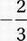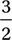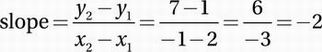# SAT Math Multiple Choice Question 607: Answer and Explanation

### Test Information

Question: 607

7. Line l intersects the graph of the function f(x) = 2x2 - 4x + 1 at two points where x = -1 and x = 2, respectively. What is the slope of line l?

• A. -2
• B.• C.• D. 2

Explanation:

A

Algebra (lines) MEDIUM

To find the slope of line l, we can find two points on l and then use the slope formula.

f(x) = 2x2 - 4x +1

Plug in -1 for x:

f(-1) = 2(-1)2 - 4(-1) + 1

Simplify:

f(-1) = 2(1) + 4 + 1 = 2 + 4 + 1 = 7

Therefore line l intersects the function at (-1, 7).

Plug in 2 for x:

f(2) = 2(2)2 - 4(2) + 1

Simplify:

f(2) = 2(4) - (8) + 1 = 8 - 8 + 1 = 1

Therefore line l intersects the function at (2, 1). Now we find the slope of the line containing these two points.